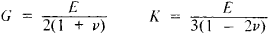# Moduli of Elasticity

The following article is from The Great Soviet Encyclopedia (1979). It might be outdated or ideologically biased.

## Moduli of Elasticity

quantities that characterize the elastic properties of materials. In the case of small deformations when the Hooke law is valid (that is, when there is a linear relationship between the stresses and strains), moduli of elasticity are the proportionality constants for the relationships.

The modulus of elongation E (Young’s modulus) corresponds in the direction of tension to a unilateral normal stress σ generated by simple tension or compression. It is equal to the ratio of the normal stress σ to the relative elongation e resulting from the stress in the direction of its action (E = σ/e) and characterizes the ability of the material to resist extension.

The shear modulus G corresponds to a pure shear stressed state in which only tangential stresses τ act on two mutually perpendicular areas. The shear modulus is equal to the ratio of the tangential stress τ to the angle of shear λ, which is determined by the distortion of the right angle between the planes upon which the tangential stresses are acting. Thus, G = τ/λ. The shear modulus determines the ability of a material to resist a change in shape while maintaining its volume. The compression modulus K, which is the bulk modulus of elasticity, corresponds to a normal stress or that is uniform in all directions (generated, for example, by hydrostatic pressure). It is equal to the ratio of the normal stress γ to the relative bulk compression A resulting from it: K γ/Δ. The bulk modulus of elasticity characterizes the ability of a material to resist a change in its volume not accompanied by a change in shape.

The Poisson ratio v is another constant that characterizes the elastic properties of materials. It is equal to the ratio of the absolute value of the compressive transverse strain of a cross section ∊′ (for unilateral extension) to the relative elongation ∊, that is, v = ǀ∊’ǀ/.

In the case of a uniform isotropic body, the moduli of elasticity are the same in all directions. The four constants E, G, K, and v are related to one another by two formulas:Thus, only two of these constants are independent quantities, and the elastic properties of an isotropic body are determined by two elastic constants.

In the case of an anisotropic material, the constants E, G, and v assume different values in different directions, and their values may vary widely. The number of moduli of elasticity of an anisotropic material depends on the structure of the material. An anisotropic body lacking any symmetry with respect to elastic properties has 21 moduli of elasticity. When symmetry is present in the material, the number of moduli of elasticity is reduced.

Moduli of elasticity are determined experimentally by mechanical testing of samples of materials. Moduli of elasticity are not rigorous constants for the same material, and they vary depending on the chemical composition of the material and its previous treatment (heat treatment, rolling, and forging). Values of moduli of elasticity also depend on the temperature of the material.

### REFERENCE

Fridman, la. B. Mekhanicheskie svoistva metallov, 2nd ed. Moscow, 1952.
The Great Soviet Encyclopedia, 3rd Edition (1970-1979). © 2010 The Gale Group, Inc. All rights reserved.
References in periodicals archive ?
Third, there were possible differences in the relation between the compressive and tensile moduli of elasticity parallel to the grain of the timber used in the tests.
We proved that such stiffness variations depend on the relation between the compressive and tensile moduli of elasticity parallel to the grain.
Leloup, "Dynamic and static moduli of elasticity of resin-based materials," Dental Materials, vol.
The moduli of elasticity of the resonance board in the x and y directions are obtained using Equation 2:
Compared to 100% wood, WPCs have lower moduli of elasticity and rupture.
(2002) evaluated the overall performance of two flexible short-tubes with two different moduli of elasticity: 7084 and 9889 kPa (1025 and 1434 psia).
Since the water/cement ratio of laterized concrete influences its strength; it can be reasonably inferred that the water/cement ratio influences the moduli of elasticity and deformability of laterized concrete at different degrees.
Comparison of dynamic moduli of elasticity E and of shear G shows an even greater excess of these values for the surface samples (Tables 2 and 3).
1(a), two moduli of elasticity, [E.sub.0] and [E.sub.c], were determined from the test.
Moduli of elasticity were determined for native and predried starch.
It is well established that in dynamic applications, heat is generated as the interface of materials with different moduli of elasticity.
The moduli of elasticity obtained by the authors using wave propagation methods and static tests confirmed that there were differences between the seven clones that were identified by the wave propagation test on the trees.

Site: Follow: Share:
Open / Close